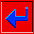## Gridding algorithms in Surface III

In the gridding procedure, a mathematical model of the surface having the form of a pattern of tilted square plates is first constructed. In simple contouring algorithms, these plates are flat. In more complex procedures they are curved, and each blends smoothly into the adjacent plates.

The mathematical model is constructed purely for practical reasons. It is much easier to draw contour lines through an array of regularly spaced grid nodes than it is to draw them through the irregular pattern of the original control points. All possible ways for a contour line to enter and leave the square grid cells can be determined in advance, and a line-drawing algorithm can easily be written to handle all possibilities. A contour line can be drawn simply by tracing the path of the line from one square of the grid to the next.

The first step in gridding by a local-fit procedure is to calculate the regular array of estimated values. Because algorithms estimate only a single location from a collection of nearby control points, the estimation procedure is repeatedly applied across the map area until the entire map area is represented by the regular grid of estimates.

The estimation process involves three essential steps. First, the control points must be sorted according to their geographic coordinates. Second, from the sorted coordinates, the control points surrounding a grid node to be estimated must be searched out. Third, the program must estimate the value of that grid node by some mathematical function of the values at these neighboring control points. Sorting greatly affects the speed of operation, and hence the cost of using a contouring program. However, this step has no effect on the accuracy of the estimates. Both the search procedure and the mathematical function do have significant effects on the form of the final map.go back